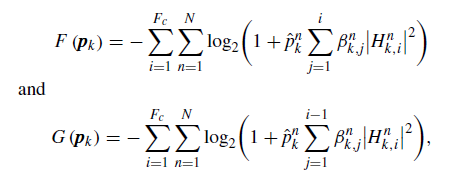# How to express accumulation expression in CVXI’m just learning to use CVX and I meet an accumulation expression , but I can’t express it in CVX , how can I solve it?

Could you help me to handle this problem?

CVX allows use of `sum`.

CVX also allows use of for loops. For instance,

``````F =  0;
for i=1:Ft
for n=1:N
for j=1:i
F = F + <whatever>;
end
end
end``````

Thanks,and I find that my error is {negative constant} ./ {convex}.Because I meet the sub expression like 1/(1+p*a),p is the variable what I want to solve,a is the constant. This expression is derived from diff.
How can I handle this problem.
If the denominator is always positive, you can use `inv_pos(1+p*a)`. I have no idea how, if at all, that relates to the formulas in your first post (for which is it not clear what is input data and what are optimization variables, nor which superscripts are powers as opposed to being index superscripts).